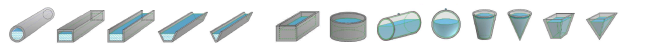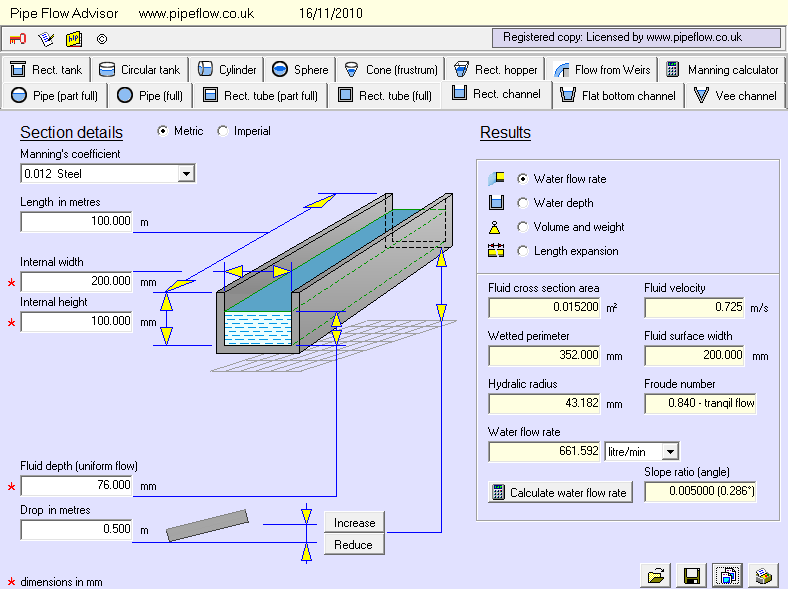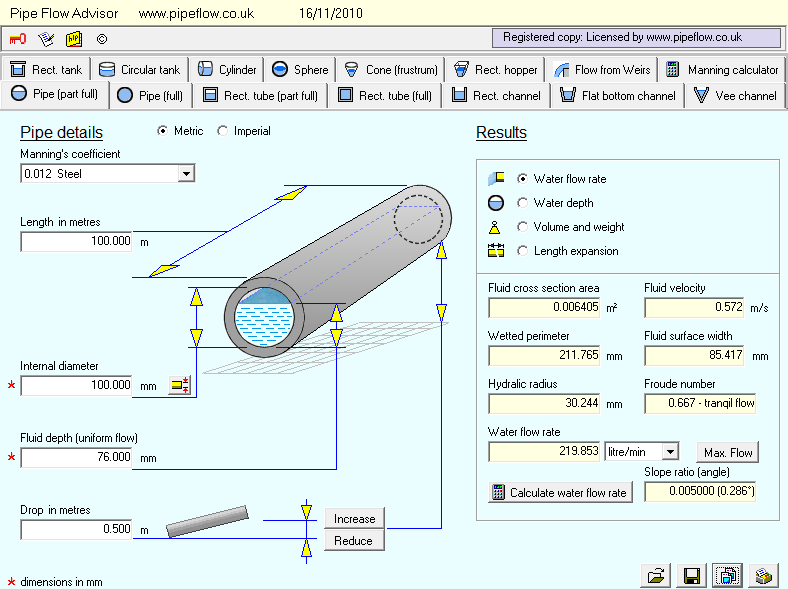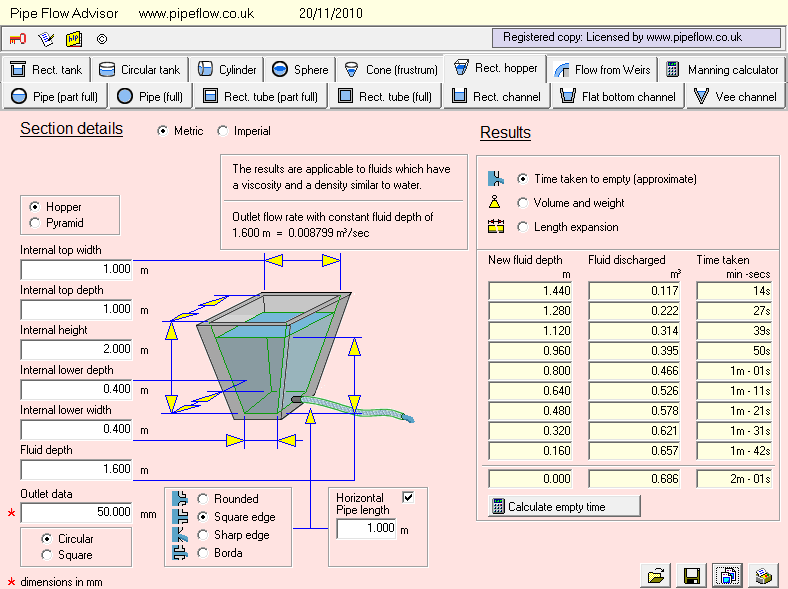Pipe Flow Advisor can estimate the flow rate of water in open channels and closed channels of different shapes. It can also estimate the flow rate from a tank, for a variety tank shapes, to calculate the tank empty time under flow due to gravity.## Flow in Open Channels, Part Full Pipes, and from TanksDo you need to calculate the flow rate in open channels?
Do you need to calculate container volumes & weight of fluid?
Do you need to find the time taken to empty a tank?

• Flow in open channels and part filled pipes
• Flow rates for weirs
• Time taken for a tank to empty with flow under gravity
• Container capacities plus volume & weight of flow in channels and pipes

## Flow in Open ChannelsFlow in an open channel on a slope occurs due to the effect of gravity on the liquid. In many cases the flow of liquid has a free surface, as in a open channel, flume or only partially full pipe. The liquid is under no pressure, other than atmospheric pressure. Over time, a number of formulae have been developed to provide an estimate of the flow rate that will occur in open-channels, with the Manning formula becoming widely accepted as the usual and best method of obtaining a reliable estimate of the flow.

## Flow in Part Full PipesThe Manning coefficient is used in the Manning formula to correct for the type of channel under consideration. The cross-sectional area of the flow, the wetted perimeter and the slope ratio must be calculated. The results are more accurate if the cross-section of flowing fluid, the fluid velocity, depth of fluid and the slope are constant (steady flow). The resulting flow rate estimates are applicable to water or fluids with similar properties to water.

## Flow from Tanks and Tank Emptying TimeThe flow rate from a tank outlet will be dependant on the head of fluid above the outlet position and the type and size of the orifice that the fluid exits through. The outlet pipe itself will also affect the flow rate, as it will introduce additional friction losses, unless it is relatively short.

As fluid exits the tank, the level of fluid remaining in the tank will decrease and thus the fluid head available to 'push' liquid out of the tank decreases over time, which in turn reduces the outlet flow rate over time. The shape of the tank also affects the tank emptying time, since for a given volume of flow out of the tank, the height of fluid lost from the tank will be different for different shapes of tank.

## Manning Coefficent and Water Flow Rate Calculation

The Manning formula (also known as the Gauckler–Manning formula) is an empirical formula based on practical experience and the results of experiments measuring flow in open channels and free-surface flow driven by gravity. It is not derived from theoretical conditions. Originally it was presented by the French engineer Philippe Gauckler in 1867 and was later re-developed in 1890 by the Irish engineer Robert Manning.

Next: Type of Channels

Secure Online PaymentsPipe Flow Software: Piping design, Pressure drop calculator, Flow rate calculator, Pump head calculations, Pump selection software.  Copyright © Pipe Flow Software 1997-2023